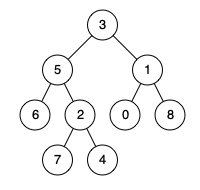# 若只如初见

0%

## 问题描述

Given the root of a binary tree, return the inorder traversal of its nodes’ values.

## 问题描述

Given a rows x cols binary matrix filled with 0’s and 1’s, find the largest rectangle containing only 1’s and return its area.

## 问题描述

Given two strings word1 and word2, return the minimum number of operations required to convert word1 to word2.

You have the following three operations permitted on a word:

Insert a character
Delete a character
Replace a character

## 问题描述

You are given n balloons, indexed from 0 to n - 1. Each balloon is painted with a number on it represented by an array nums. You are asked to burst all the balloons.

If you burst the ith balloon, you will get `nums[left] * nums[i] * nums[right]` coins. Here left and right are adjacent indices of i. After the burst, the left and right then becomes adjacent.

## 问题描述

Given a positive integer n, find the least number of perfect square numbers (for example, 1, 4, 9, 16, …) which sum to n.

Example 1:

## 问题描述

Given a binary tree, find the lowest common ancestor (LCA) of two given nodes in the tree.

According to the definition of LCA on Wikipedia: “The lowest common ancestor is defined between two nodes p and q as the lowest node in T that has both p and q as descendants (where we allow a node to be a descendant of itself).”

Example 1:## 问题描述

Given a binary search tree (BST), find the lowest common ancestor (LCA) of two given nodes in the BST.

According to the definition of LCA on Wikipedia: “The lowest common ancestor is defined between two nodes p and q as the lowest node in T that has both p and q as descendants (where we allow a node to be a descendant of itself).”

Example 1:

## 问题描述

Given a binary tree, return the zigzag level order traversal of its nodes’ values. (ie, from left to right, then right to left for the next level and alternate between).

## 问题描述

The thief has found himself a new place for his thievery again. There is only one entrance to this area, called the “root.” Besides the root, each house has one and only one parent house. After a tour, the smart thief realized that “all houses in this place forms a binary tree”. It will automatically contact the police if two directly-linked houses were broken into on the same night.

Determine the maximum amount of money the thief can rob tonight without alerting the police.

## 问题描述

Given a string that contains only digits 0-9 and a target value, return all possibilities to add binary operators (not unary) +, -, or * between the digits so they evaluate to the target value.